# Predictions of General Relativity

Back to General Relativity

#### Orbit of Mercury 1859

• A planet’s orbit precesses (see diagram) due to the gravitational attraction of nearby planets. Newton’s Theory of Gravitation was able to predict the precession of every planet except one.
• In 1859 Urbain Le Verrier of France calculated that Newton’s theory could predict 93% of Mercury’s precession. He proposed the existence of another planet, Vulcan, to explain the missing 7%.
• The perihelion of Mercury’s orbit precesses at the rate of 5599.74 arcseconds per century (relative to Earth vs ICRF). Newton’s theory was able to explain 5557 arcseconds, leaving 43 arcseconds unexplained. GR nailed the prediction. (A 1913 version of GR had gotten the prediction wrong.)
• A degree º is 1/360 of a turn around a circle
• An arcminute ′ is 1/60 of a degree.
• An arcsecond ″ is 1/60 of an arcminute.
• So an arcsecond is 1/1,296,000 of a turn.

#### Gravitational Deflection of Light 1919

• General Relativity predicts that massive bodies like the Sun make nearby spacetime so curved that light rays passing by are deflected.
• In 1919 English astronomer Sir Arthur Eddington verified this prediction by observing a star behind the Sun during a total eclipse.  Light from the star was deflected 1.75 seconds of arc, as predicted by General Relativity.
• Einstein became world famous.

#### Gravitational Time Delay of Light 1964 and 1976

• General Relativity predicts that light rays passing by massive bodies are delayed because the rays, bent by the body, travel a greater distance and therefore take longer.
• The time delay was confirmed in 1976 by bouncing radar signals off the radar transponders of Viking landers on Mars.

#### Gravitational Time Dilation 1971

• Gravitational Time Dilation
• General Relativity predicts that time runs slower in a gravitational field.
• Kinetic Time Dilation
• Special Relativity predicts that time runs slower in a reference frame moving relative to another.
###### Hafele-Keating Experiment
• In 1971 J.C. Hafele and R. E. Keating flew four atomic clocks on commercial jets around the world twice, once eastward (41 hours), once westward (49 hours).
• Based on both Special Relativity and General Relativity they predicted the following nanosecond increases on the flying clocks relative to clocks on Earth:
• The best atomic clocks are predicted to be off by less than one second in 50 million years.
###### Gravitational Time Dilation Calculation
• You are at the Black Dot, far away from the Big Sphere. You define a reference frame with spherical coordinates.  The r axis is the dashed line, with r = 0 at the center of the Big Sphere. You keep time with an atomic clock at the Black Dot.
• The Blue Dot on the surface of the Big Sphere is at space coordinates (r, 𝛳, 𝜙), where r = the radius of Earth, 𝛳 = 0, and 𝜙 = 0.
• Two light flashes take place at the Blue Dot about a day apart. An atomic clock at the Blue Dot measures the proper time interval d𝝉 between flashes.
• The proper time 𝝉 along a worldline is the time measured by a clock carried along the worldline.
• You want to calculate the proper time d𝝉 between flashes.
• The line element of the Schwarzschild Metric defines spacetime for (ct, r, 𝜽, 𝝓)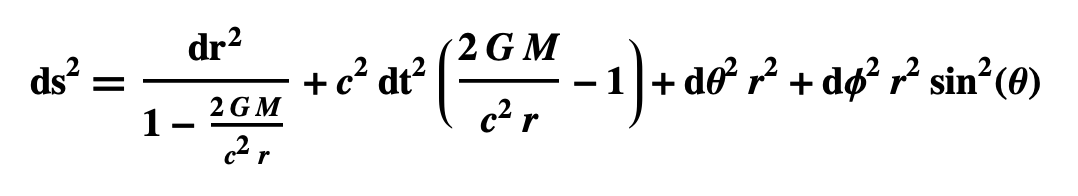• The square of the proper time interval is defined as: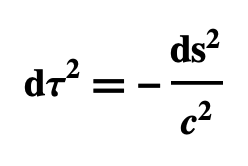• Putting the equations together, we get: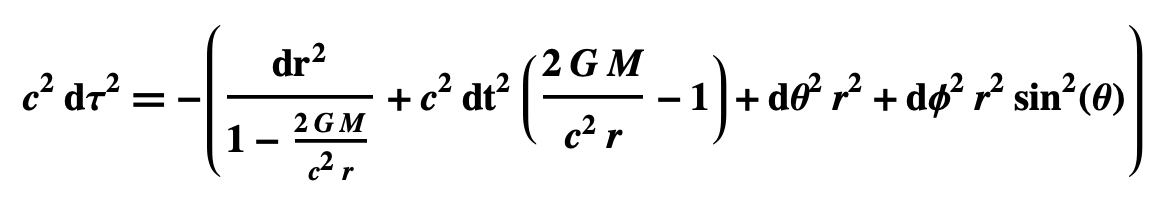• dr, d𝛳, and d𝜙 represent the difference in location between the light flashes at the Blue Dot.  Since the flashes occur at the same location, dr = 0, d𝛳 = 0, and d𝜙 = 0. This reduces the equation to: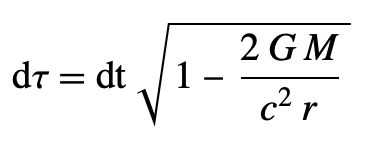• The proper time interval d𝜏 is the time interval measured by a clock at the Blue Dot.
• The coordinate time interval dt is the time measured by your clock at the Black Dot.
• Assume
• M = Earth’s mass 5.972 x 1024 kg
• r = Earth’s radius 6.37814 x 106 m
• Then for dt = 86,400 seconds (a day’s worth),  d𝜏 = 86399.999940 seconds.
• Time thus runs slower in a gravitational field.
###### Global Positioning System and Time Dilation

The GPS system incorporates both Special and General Relativity. Otherwise it wouldn’t work.

#### Gravitational Waves 2015

• In his 1918 paper “On Gravitational Waves” Einstein predicted the existence of gravitational waves, detected in 2015 by interferometers in Louisiana and Washington state.
• A gravitational wave is a wave of expanding and contracting space traveling at the speed of light.
• A gravitational wave passing through a ring of particles expands and contracts space so that the particles deform vertically into an ellipse, back to a circle, horizontally into an ellipse, back to a circle, and so on.
• Time 1: Space is normal
• Time 2: Space contracts horizontally and expands vertically.
• Time 3: Space is normal again
• Time 4: Space expands horizontally and contracts vertically.
• In the diagrammed interferometer, a laser shoots a beam toward the half-transparent mirror (HTM).  The A-half of the beam passes through the HTM and is reflected by the fixed mirror; half the returning beam is reflected by the HTM onto the Screen. The B-half of the beam is reflected by the HTM and bounces off the moveable mirror; half the returning beam passes through the HTM to the Screen. If the A and B beams travel precisely the same distance, their waves will be in phase at the Screen and interfere constructively.
• Before Einstein put forth Special Relativity in 1905, physicists thought the speed could differ depending on direction. In 1887 Michelson and Morley used an interferometer to show that light rays at right angles to each other travelled at the same speed.
• Two LIGOs (Laser Interferometer Gravitational-Wave Observatories) detected a gravitational wave on Sep 14, 2015, first at the LIGO in Louisiana and 7 milliseconds later at the LIGO in Washington.
• Each LIGO arm is a 2.5 mile-long evacuated tube, 4 feet in diameter, through which a laser beam passes.
• As the wave passed through the LIGOs in September, space in the arms alternately expanded and contracted, causing the laser beam to travel different distances.
• The wavelength was about a million meters
• The cause was two black holes spiraling into one another 1.3 billion years ago.

View Britannica Video (begin at 2:20)

#### Big Bang Theory 1922 and 1929

• Aleksandr Friedmann (1922), Howard P. Robertson (1929), and Arthur G. Walker (1929) solved Einstein’s Field Equation for a universe that’s homogeneous (of the same density everywhere) and isotropic (the same in all spatial directions). The Friedmann-Robertson-Walker metric provides the theoretical basis for the Big Bang Theory, predicting the age, expansion, and fate of the universe.
• FRW Metric: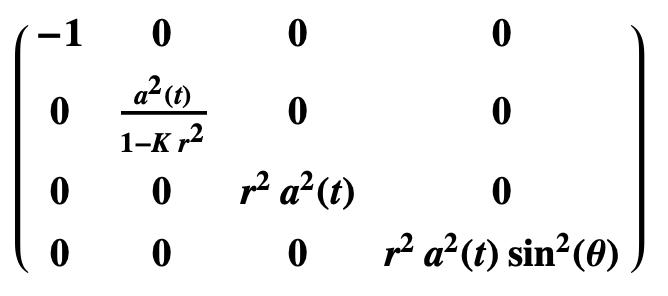• FRW Spacetime Interval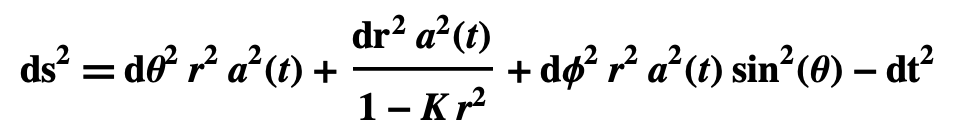View Big Bang Theory

#### Gravitational Redshift 1959

• GR predicts that light is redshifted as it travels away from a massive object and blueshifted as it approaches.
• The phenomenon is the result of Gravitational Time Dilation
• Five waves per a long second near a massive object means higher frequency and a shift toward blue
• Four waves per a longer second far away from the massive object means lower frequency a shift toward red

#### Gravitational Lensing 1979

• britannica.com/science/gravitational-lens
• “In the 1930s Einstein predicted that a mass distribution, such as a galaxy, could act as a gravitational “lens,” not only bending light but also distorting images of objects lying beyond the gravitating mass. If some object is behind a massive galaxy, as seen from Earth, deflected light may reach Earth by more than one path. Operating like a lens that focuses light along different paths, the gravity of the galaxy may make the object appear stretched or as though the light is coming from multiple objects, rather than a single object. The object’s light may even be spread into a ring.”
• “The first gravitational lens was discovered in 1979, when two quasars were discovered very close to each other in the sky and with similar distances and spectra. The two quasars were actually the same object whose light had bee

Gravitational Lensing Hubble Photo 1994

• britannica.com/science/gravitational-lens
• “In this picture a galactic cluster, about five billion light-years away, produces a tremendous gravitational field that “bends” light around it. This lens produces multiple copies of a blue galaxy about twice as distant. Four images are visible in a circle surrounding the lens; a fifth is visible near the centre of the picture.”
• hyperphysics.phy-astr.gsu.edu/hbase/Astro/quasar.html#c3
• “The cluster is 5 billion light-years away in the constellation Pisces, and the blue-shaped galaxy is about 2 times farther away.” When the amount of bending is analyzed, the required bending mass is greater than can be accounted for from the visible mass of the cluster, so this is another piece of evidence for the existence of dark matter.”

#### Frame Dragging and Geodetic Effect 2005

• Suppose a gyroscope is in orbit around a non-rotating sphere, with the axis of the gyroscope pointed to a faraway star.
• Both Newton’s theory and GR predict that the spin of the gyroscope will remain constant as it orbits.  But GR makes a different prediction if the sphere is rotating, like the Earth. Due to what are called Frame-dragging and the Geodetic Effect, GR predicts the gyroscope will precess.
• britannica.com/topic/Gravity-Probe-B
• “The mission of the Gravity Probe B Spacecraft, launched April 20, 2004 into polar orbit, was to test GR’s predictions of frame-dragging (by which the rotation of the Earth slowly drags spacetime around it as it rotates) and the geodetic effect (by which the Earth’s curvature of spacetime causes the circumference of an orbit to be smaller than it would be if spacetime were flat).  The craft carried four gyroscopes of ultraprecision 4-cm  polished quartz spheres spinning in liquid helium. The mission of GP-B ended on Sept. 25, 2005. The measured values for these effects agreed with those predicted by general relativity.”
• Photo of Probe